Normal view MARC view ISBD view

# Exploring linear algebra: labs and projects with mathematica

Series: Textbooks in Mathematics. Publisher: Boca Raton CRC Press 2015Description: viii, 139 p.ISBN: 9781482241495.DDC classification: 512​.5028553 Summary: Exploring Linear Algebra: Labs and Projects with Mathematica® is a hands-on lab manual for daily use in the classroom. Each lab includes exercises, theorems, and problems that guide your students on an exploration of linear algebra. The exercises section integrates problems, technology, Mathematica® visualization, and Mathematica CDFs, enabling students to discover the theory and applications of linear algebra in a meaningful way. The theorems and problems section presents the theoretical aspects of linear algebra. Students are encouraged to discover the truth of each theorem and problem, to move toward proving (or disproving) each statement, and to present their results to their peers. Each chapter also contains a project set consisting of application-driven projects that emphasize the material in the chapter. Students can use these projects as the basis for further undergraduate research. (https://www.crcpress.com/Exploring-Linear-Algebra-Labs-and-Projects-with-Mathematica-/Arangala/9781482241495)
Tags from this library: No tags from this library for this title.
Item type Current location Item location Collection Call number Status Date due Barcode
Books Vikram Sarabhai Library
Slot 1364 (0 Floor, East Wing) Non-fiction 512​.5028553 A7E9 (Browse shelf) Available 190115
##### Browsing Vikram Sarabhai Library Shelves , Collection code: Non-fiction Close shelf browser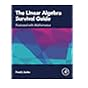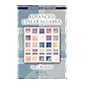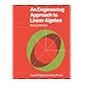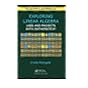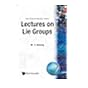512.5 S9L4 The linear algebra survival guide: illustrated with mathematica 512.5 W6A2 Advanced linear algebra 512.502462 S2E6 An engineering approach to linear algebra 512​.5028553 A7E9 Exploring linear algebra: labs and projects with mathematica 512.55 H2L4 Lie groups, lie algebras, and representations: an elementary introduction 512.55 H8L3 Lectures on Lie groups 512.55 S4O7 Operator theory: a comprehensive course in analysis, part 4

1. Matrix Operations
2. Invertibility
3.Vector Spaces
4.Orthogonality
5.Matrix Decomposition with Applications
6.Applications to Differential Equations
7.Mathematica Demonstrations and References

Exploring Linear Algebra: Labs and Projects with Mathematica® is a hands-on lab manual for daily use in the classroom. Each lab includes exercises, theorems, and problems that guide your students on an exploration of linear algebra. The exercises section integrates problems, technology, Mathematica® visualization, and Mathematica CDFs, enabling students to discover the theory and applications of linear algebra in a meaningful way. The theorems and problems section presents the theoretical aspects of linear algebra. Students are encouraged to discover the truth of each theorem and problem, to move toward proving (or disproving) each statement, and to present their results to their peers. Each chapter also contains a project set consisting of application-driven projects that emphasize the material in the chapter. Students can use these projects as the basis for further undergraduate research.

(https://www.crcpress.com/Exploring-Linear-Algebra-Labs-and-Projects-with-Mathematica-/Arangala/9781482241495)

There are no comments for this item.